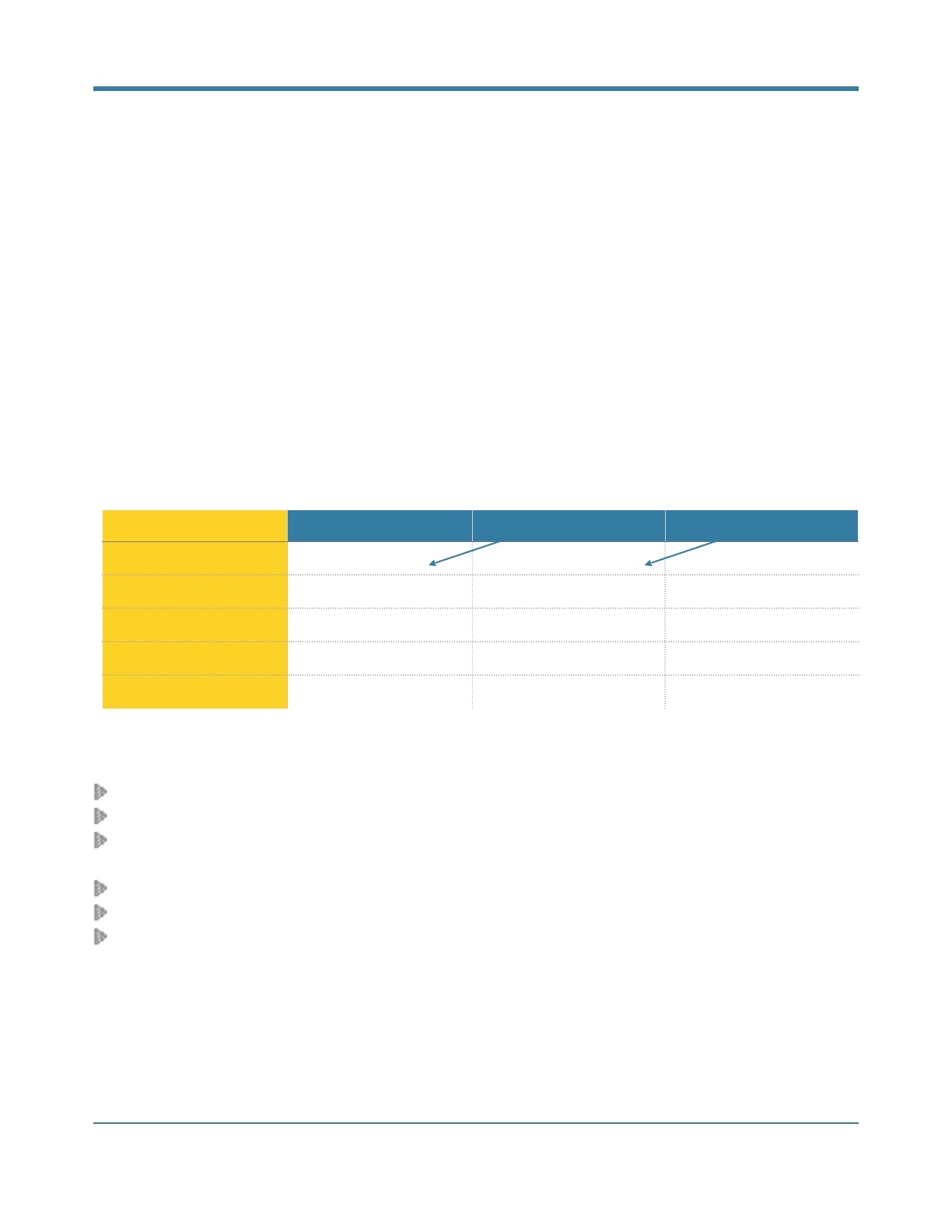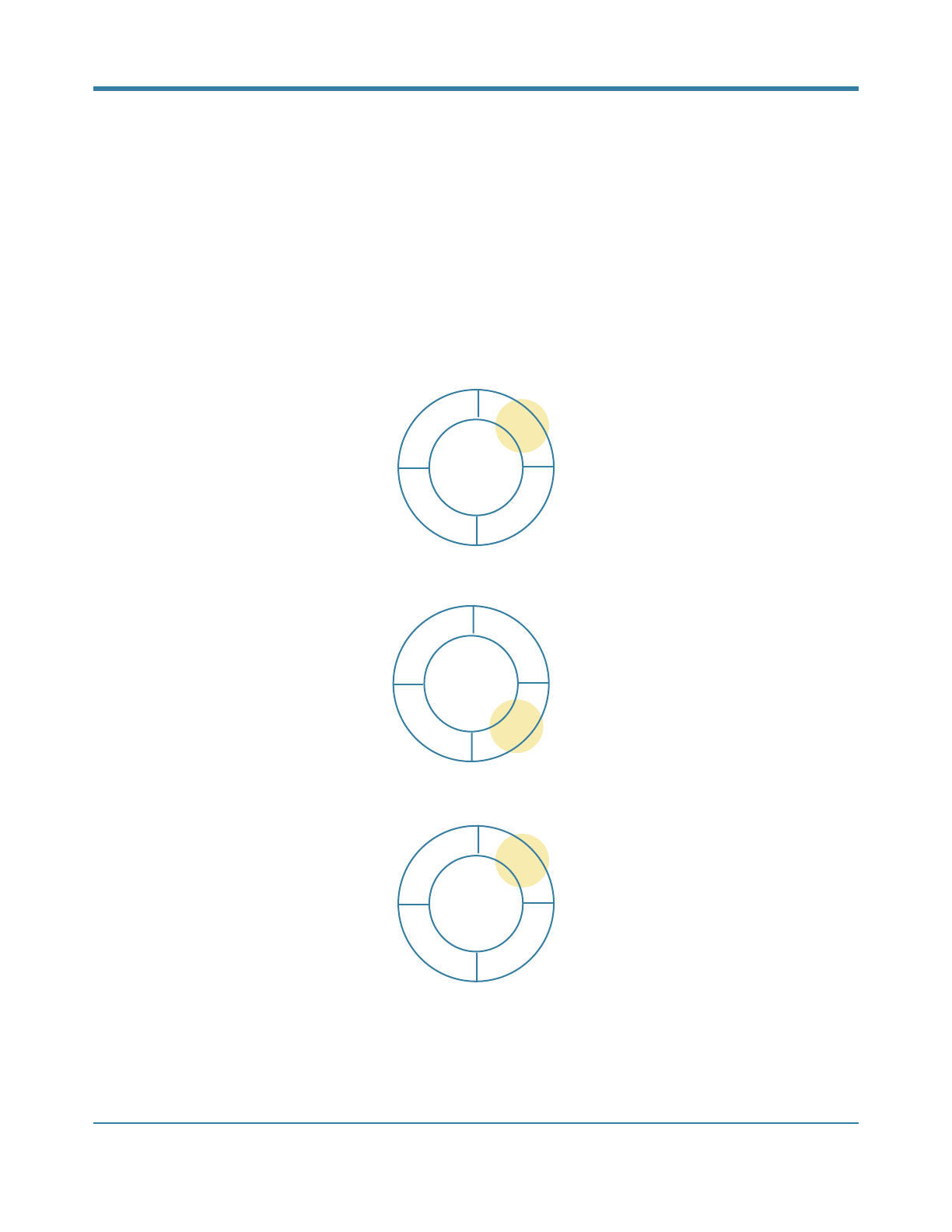Fibonacci Microtonal Music
Lilac Atassi
May 14, 2016
INTRODUCTION
This article explains how the Fibonacci sequence is used to produce the microtonal music streamed live at https://
www.youtube.com/c/LilacAtassi/live. The computer program can produce 24 notes with fundamental frequencies
from 261.6 Hz to 508.3 Hz, the distance between two adjacent notes is 50 cents. The modulo operation is used
to emulate a circular instrument in effect, as if the last and ﬁrst keys of the piano are next to each other.
Starting from the ﬁrst note, the Fibonacci numbers tell how many notes should be skipped to get to the note that
should be played. The same approach is used to also select the duration of each note. The one-step delayed Fi-
bonacci numbers select a rhythm from the 24 predeﬁned values, 40 to 270 bpm with 10 bpm intervals, which is
then used to set the note duration.
The explained procedure leads to a surprisingly unique composition. The amount of time it takes before a group of
5 notes are repeated can be estimated. As there are 24 notes to choose from and 24 durations, there are 576
(24×24) options for each note. Therefore, a group of 5 notes has 576
5
possible arrangements. Let’s assume each
note is played for 0.5 seconds on average. Then it would take more than 1 million years to hear a repeated group
of 5 notes.
The next two sections introduce the Fibonacci sequence and the modulo operation in greater details.
!1FIBONACCI SEQUENCE
The Fibonacci sequence is deﬁned by the following recursive equation
F
n+2
=
F
n
+
F
n+1
for n 0, F
1
= 0 and F
2
= 1
This is a recursive equation because to calculate say the tenth number, F
10
, the values of F
9
and F
8
are needed. It
is also possible to compute the values going forward. First the values of F
1
and F
2
are computed to get the value
of F
3
. The following table shows how to calculate the values.
An easy way to implement this is to deﬁne three variables, X, Y, and Z.
First initialize X and Y, X = F
1
= 0 and Y = F
2
= 1. Then the algorithm continues:
calculate the value of Z
= X + Y
= 0 + 1 = 1.
update the value of X, X = Y = 1.
update the value of Y, Y = Z = 1.
Then the second iteration would be (the second row in the table):
calculate the value of Z = X + Y = 1 + 1 = 2.
update the value of X, X = Y = 1.
update the value of Y, Y = Z = 2.
This set of operations can be repeated forever. The above table shows the results of the ﬁrst 5 iterations. Each of
the X, Y, and Z columns contains the Fibonacci numbers, with X being one step behind Y, and Y one step behind
Z.
In the Fibonacci Microtonal Music, the numbers in the Y column are used to select the notes, and the numbers in
the X column are used to select the note durations.!
Step Number
X
Y
Z
1
0
1
2
1
2
3
1
3
4
2
5
5
3
8
!2
FIBONACCI MICROTONAL MUSICMODULO OPERATOR
To select a note and tempo using the Fibonacci numbers the module operator is used. In the programming lan-
guages % is used modulo operator. The operator calculates the remainder of an integer division. 4 % 2 is zero,
and 5 % 2 is 1, for instance.
To see how modulo is used, assume there are 4 containers. An object from the ﬁrst container can be selected.
Then can go 1 container ahead and take an object from that container. That would be the second container.
At this stage, can go ahead 3 containers. That’s the reason the containers are arranged in a circle. Taking 3 steps,
arrives back in the container number 1.
When at number 2, after taking 3 steps, that is 2 + 3 = 5, but there are only four containers. The modulo operator
gives the right number, (2+3) % 4 = 1. Therefore, this equation can be used for this simple example:
[next container] = ( [current container] + [number of steps] ) % [number of containers].
!3
FIBONACCI MICROTONAL MUSIC
1
2
3
4
1
2
3
4
1
2
3
4